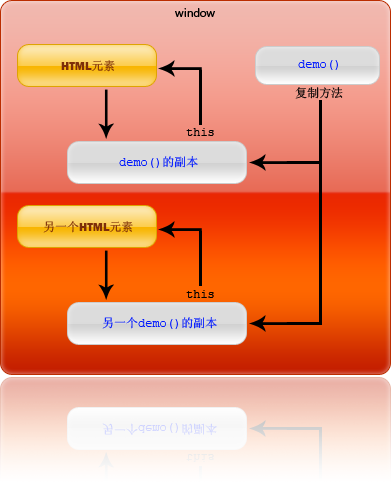# JS删除数组里的某个元素方法_javascript技巧js删除数组中某一项或几项的几种方法 https://www.zgxue.com/article/154737.htm

String[]arrays={\"1\",\"2\",\"3\",\"5\",\"6\"};String[]tempArr=new String[arrays.length];int i=0;for(String s:arrays){ 数组本身是不可以移除元素的 但可以通过中间变量来实现数组的移除delete和splice都可以删除数组中的元素，但是有些区别的，我举具体的例子给你看，你看后就明白了。例如有一个数组是：var textArr=['a','b','c','d'];这时我想删除这个数组中的b元素： 方法一：Array.prototype.indexOf = function(val) { for (var i = 0; i < this.length; i++) { if (this[i] == val) return i; } return -1; };

Array.prototype.remove=function(dx){ if(isNaN(dx)|dx>this.length){ return false;} for(var i=0,n=0;i;i+){ if(this[i]!this[dx]){ this[n+]=this[i] } } this.length-=1 }Array.prototype.remove = function(val) { var index = this.indexOf(val); if (index > -1) { this.splice(index, 1); } };

var emp = ['abs','dsf','sdf','fd']

emp.remove('fd');

splice(index,len,[item]) 注释：该方法会改变原始数组。

splice有3个参数，它也可以用来替换/删除/添加数组内某一个或者几个值

index:数组开始下标 len: 替换/删除的长度 item:替换的值，删除操作的话 item为空

//删除起始下标为1，长度为1的一个值(len设置1，如果为0，则数组不变) var arr = ['a','b','c','d']; arr.splice(1,1); console.log(arr); //['a','c','d']; //删除起始下标为1，长度为2的一个值(len设置2) var arr2 = ['a','b','c','d'] arr2.splice(1,2); console.log(arr2); //['a','d']

//替换起始下标为1，长度为1的一个值为‘ttt'，len设置的1 var arr = ['a','b','c','d']; arr.splice(1,1,'ttt'); console.log(arr); //['a','ttt','c','d'] var arr2 = ['a','b','c','d']; arr2.splice(1,2,'ttt'); console.log(arr2); //['a','ttt','d'] 替换起始下标为1，长度为2的两个值为‘ttt'，len设置的1

var arr = ['a','b','c','d']; arr.splice(1,0,'ttt'); console.log(arr); //['a','ttt','b','c','d'] 表示在下标为1处添加一项'ttt'

delete方法删除掉数组中的元素后，会把该下标出的值置为undefined,数组的长度不会变

var arr = ['a','b','c','d']; delete arr; arr; //["a", undefined × 1, "c", "d"] 中间出现两个逗号，数组长度不变，有一项为undefined

• 本文相关：
• javascript之高级数组api的使用实例
• 详解js数组reduce()方法详解及高级技巧
• 深入javascript高级程序设计之对象、数组（栈方法，队列方法，重排序方法，迭代方法）
• js删除数组元素、清空数组的简单方法(必看)
• js数组循环遍历数组内所有元素的方法
• js array 数组详解
• js二维数组定义和初始化的三种方法总结
• js数组去重的三种常用方法总结
• javascript从数组中删除指定值元素的方法
• js数组的高级使用方法示例小结
• 在原生不支持的旧环境中添加兼容的object.keys实现方法
• js统计flash被网友点击过的代码
• javascript建立一个语法高亮输入框实现思路
• js图片向右一张张滚动效果实例代码
• 微信小程序实现跑马灯效果
• js replace替换所有匹配的字符串
• javascript类型系统_正则表达式regexp类型详解
• es6 iterator接口和for...of循环用法分析
• javascript实现点击出现图片并统计点击次数功能示例
• js 怎么在数组删除第一个元素
• js数组元素怎样删除最后一个元素
• js如何删除数组里的某个元素
• js中怎么将数组中某个元素去掉
• js从数组中删除指定值（不是指定位置）的元素
• java如何删除掉数组中的某个元素？？
• js删除数组中的元素delete和splice的区别
• 在JS数组中如何删除某个元素
• js数组删除所有重复的元素
• js 怎样删除数组中的最后一个元素并返回第一个元素?
• 免责声明 - 关于我们 - 联系我们 - 广告联系 - 友情链接 - 帮助中心 - 频道导航